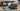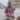Posted November 16, 2016Start discussion

I like reading short and expressive code. Because developer spends more time reading code than writing, expressiveness often is obligatory.

Unless shortness does not obscure the intent, I favor concise expressions over longer ones. For example:

`swift`import Foundationlet greeting = "Hello, "  let who = "World"let message1 = greeting + who  let message2 = greeting.appending(who)  print(message1) // => "Hello, World"  print(message2) // => "Hello, World"  ``

The sample shows 2 options to concatenate strings: using addition operator `+` or `appending(_)` string method.
What option do you like more? I guess the concise one `greeting + who`.

The operator overloading in Swift enables to write short expressions. Many types like `Int`, `String`, `Array` overload addition (`+`) and addition assignment (`+=`) operators. It makes the manipulation of the corresponding types more intuitive.

Would you like to write expressive code? I’m sure you do! So let’s continue with an interesting list of types that support operator overloading for `+` and `+=`.
The alternative methods with the same behavior are also presented, for comparison purposes.

## 1. Sum numbers

Obviously the regular usage of addition operator is meant to perform arithmetic addition on numbers. For instance, `4 + 8` is evaluated to `12`.

Because Swift is type-safe, you can apply `+` and `+=` operators when both operands are exactly the same type (`Int + Int`, but not `UInt + Int` or `Float + Int`).

All Swift number types `Int`, `Float`, `Double` and others support addition operators. Let’s see a sample:

`swift`var x = 5let y = 3print(x + y) // => 8x += yprint(x)     // => 8``

`x + y` performs an arithmetic addition of two integers. Plain and simple.
The expression `x += y` mutates `x` variable by appending `y` to it (same as `x = x + y`). During this operation `x` is mutated, so it must declared as a variable with `var`.

The equivalent methods of addition operators are `adding(_:)` and the mutating `add(_:)`. These methods are available for `Float` and `Double`, but not for `Int`.
Let’s see them in action:

`swift`var p = 5.0let r = 3.0print(p.adding(r)) // => 8.0p.add(r)print(p)           // => 8.0``

`p` and `r` are `Double` type.
The invocation of `p.adding(r)` is the same as `p + r`. Respectively `p.add(r)` mutates `p` and is equivalent to `p += r`.

## 2. Concatenate strings

The addition and addition assignment operators can perform strings concatenation. For instance, `"abc" + "def"` creates a string `"abcdef"`.

Let’s see an example:

`swift`var message = "Hello "let name = "Batman"print(message + name) // => "Hello Batman"message += nameprint(message)        // => "Hello Batman"``

`message + name` concatenates two strings.
The statement `message += name` also performs a concatenation. It modifies `message` in place by appending to its end `name` string.

You can also use equivalent methods `appending(_:)` and mutating `append(_:)`, which are more verbose. Let’s transform the above example:

`swift`var message = "Hello "let name = "Batman"print(message.appending(name)) // => "Hello Batman"message.append(name)print(message)                 // => "Hello Batman"``

The invocation `message.appending(name)` concatenates `message` and `name`, returning the result. `message` variable is not modified.
The invocation `message.append(name)` is mutating the `message` variable and appends to its end `name` string.

## 3. Concatenate arrays

Addition operators are useful to concatenate arrays. `[val1, val2] + [val3]` creates `[val1, val2, val3]`.

The concatenated arrays must have elements of the same type.

`swift`var colors = ["white"]let darkColors = ["black", "gray"]print(colors + darkColors) // => ["white", "black", "gray"]colors += darkColorsprint(colors)              // => ["white", "black", "gray"]``

The expression `colors + darkColors` creates a new array that contains elements from `colors` followed by elements from `darkColors`.
`colors += darkColors` mutates the `colors` array in place, by adding to its tail elements from `darkColors`.

Alternatively you can use the mutating `append(contentsOf:_)`, which is an equivalent of addition assignment operator (`+=`). Transforming the above example:

`swift`var colors = ["white"]let darkColors = ["black", "gray"]colors.append(contentsOf: darkColors)print(colors) // => ["white", "black", "gray"]``

The invocation of `colors.append(contentsOf: darkColors)` modifies `colors` in place and appends the elements of `darkColors`.

## 4. Add time interval to a date

The addition operator enables expressively to add intervals to a `Date`. The expression `date + timeInterval` creates a new `Date` with a specified amount of time added to it. The addition assignment modifies the date in place `date += timeInterval`.

Let’s see how it can be done:

`swift`import Foundationlet interval: TimeInterval = 60 * 60 * 24let dateFormatter = DateFormatter()dateFormatter.dateFormat = "yyyy-MM-dd"if let date = dateFormatter.date(from: "2017-02-15") {   let dayAfter = date + interval      print(dateFormatter.string(from: dayAfter)) // => 2017-02-16}``

`oneDay` is an interval that contains the number of seconds in 24 hours. `dateFormatter` creates a date for `2017-02-15`.
The expression `date + oneDay` evaluates to a new date `dayAfterDate` that is created from `date` with `oneDay` time interval added to it.

If you want to modify `date` directly, make it a variable and use addition assignment operator `+=`:

`swift`/* ... */if var date = dateFormatter.date(from: "2017-02-15") {   date += interval      print(dateFormatter.string(from: date)) // => 2017-02-16}``

`date =+ oneDay` mutates `date` by adding `oneDay` seconds to it.

The `Date` methods that provide the same behavior are `addingTimeInterval(_:)` and the mutating `addTimeInterval(_:)`.

The provided way to modify a date adjusts absolute values. You may have unexpected results when adding longer time intervals like weeks or months.

Most of the times `Calendar` usage is preferable. It provides accurate date modifications according to daylight saving time, months with different numbers of days, and so on.

Let’s update the above example and use a calendar instance `Calendar.current`:

`swift`import Foundationlet interval = 60 * 60 * 24let dateFormatter = DateFormatter()dateFormatter.dateFormat = "yyyy-MM-dd"if let date = dateFormatter.date(from: "2017-02-15") {   let calendar = Calendar.current   let dayAfter =      calendar.date(byAdding: .second, value: interval, to: date)!   print(dateFormatter.string(from: dayAfter)) // => 2017-02-16}``

## 5. Sum measurements

A recent Foundation update introduced measurements and units. It allows to represent distances (for instance `10 miles`, `12 kilometers`), weights (`8 kg`) and more.

The good part is that `Measurement` structure overloads `+` operator (and additionally `* - / < ==`). This makes measurement manipulations easy and concise.

Let’s sum two distances in kilometers:

`swift`import Foundationlet morningRun = Measurement(value: 3, unit: UnitLength.kilometers)let eveningRun = Measurement(value: 5, unit: UnitLength.kilometers)let dailyRun = morningRun + eveningRunprint(dailyRun) // => '8000.0 m'``

`morningRun` and `eveningRun` describe the distance someone ran in the morning and evening.
Plain and simple the addition operator is used to find the daily run distance: `morningRun + eveningRun`.

The addition operation must sum measurements that describe the same type of physical units (length, mass, speed and more).

For instance, it doesn’t make sense to sum speed and mass values. In such case Swift triggers an error:

`swift`import Foundationlet turtleSpeed = Measurement(value: 3, unit: UnitLength.kilometers)let turtleWeight = Measurement(value: 100, unit: UnitMass.grams)print(turtleSpeed + turtleWeight) // Error: binary operator '+' cannot be applied to operands of // type 'Measurement<UnitLength>' and 'Measurement<UnitMass>'``

`turtleSpeed` and `turtleWeight` are measurements that holds different type of units: `UnitLength.kilometers` and `UnitMass.grams`. These are not compatible, and as result Swift triggers an error.

`Measurement` structure does not provide methods for manipulation. In this case you have to use operators only.
In my opinion it’s a nice decision, because operators fits good with measurements.

## 6. Conclusion

As seen, the addition and addition assignment operators provide short and concise syntax.

Generally these are used to sum numbers and concatenate strings.

You can also benefit from a concise syntax when concatenating arrays, manipulating dates and sum measurements.

Do you know other Swift types that implement addition operator overloading? Feel free to write a comment below!

## Quality posts into your inbox

I regularly publish posts containing:

• Important JavaScript concepts explained in simple words
• Overview of new JavaScript features
• How to use TypeScript and typing
• Software design and good coding practices